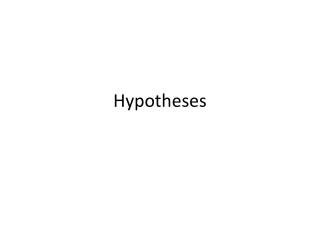# Hypotheses - PowerPoint PPT PresentationDownload PresentationHypotheses

Hypotheses
Download Presentation## Hypotheses

- - - - - - - - - - - - - - - - - - - - - - - - - - - E N D - - - - - - - - - - - - - - - - - - - - - - - - - - -
##### Presentation Transcript

1. Hypotheses

2. Hypotheses are about applying theory to your research question • A good research paper starts with a context that conceptualizes the problem, often with formal theory, but at least with heuristics. • The crux of research is applying theory to shed light on some problem.

3. Conceptualizing problems is hard to teach • It is abstract • It requires insight and understanding of the problem • It requires synthesis of concepts and “real world” aspects of the problem • It requires a strong foundation in economic theory and the application of economic theory to applied problems • Should structure your concept of your problem around major economic theories • Eg, utility maximization, rational expectations, etc.

4. Class participation exercise • Someone please propose one of their research questions • Let’s have a discussion about what theories might be relevant to this question • We’ll do this for several of the questions

5. Theorizing is simply thinking about an issue in the context of economic theories and analysis, mostly to identify how they relate. • Ask yourself three questions • What are the essential concepts involved in the problem being researched? • How are these essential concepts related? • What implications or predictions can be drawn from these relationships? • Theory can be narrative (conceptual) or mathematical. You can build a model from scratch, but more usual you will borrow and adapt an existing model from the literature.

6. Class participation exercise • For the questions we just discussed, let’s to some theorizing • Use the context of the three questions • Does anyone know existing models that might apply? • We’ll do this for several of the questions

7. Good theorizing leads to testable hypotheses • A hypothesis • is an educated guess about how things work.; • is a prediction about the outcome of the research. ;is the proposed answer to the research question or the main assertion about the topic; • will be supported by the rest of the paper. • A good hypothesis provides a framework around which a paper can be organized.

8. Your hypothesis • Should be stated simply and clearly; • Must be able to be separated from alternative hypotheses; • Primary hypothesis and alternative hypothesis must not overlap. • They must be clearly distinguishable, • It should be testable, to be proven false. • It should depend on the theory. • If the test is empirical, the data for the test should be available or at least possible.

9. Class participation exercise • For the theories we just discussed, let’s develop some hypotheses • Let’s evaluate them for being “good” hypotheses. • We’ll do this for several of the questions

10. The hypothesis and the research process • Question • Your hypotheses should answer the question. You may want to start with an If…then statement. • You may need to examine more than one hypothesis to address all aspects of the question • Theory – the hypothesis is derived from theory • Model – the hypothesis appears most likely at the end of the model section as the testable result of this section. • Analysis – the test of the hypothesis • Results – support or falsify the hypothesis. • Implications/conclusions – discuss what the hypothesis means, why the result is important and possibly alternative hypotheses

11. Class participation • Let’s go back to the examples, and explore how the hypotheses relate to the question, and the rest of the research process.